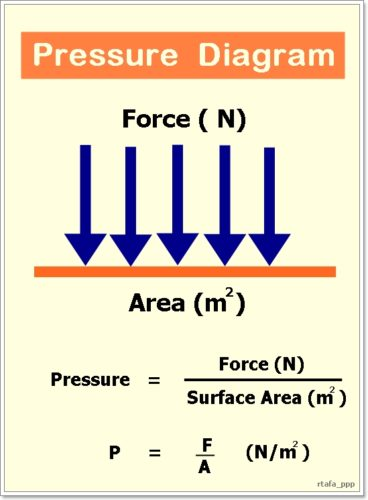# Pressure | Types | Classification of Measurement Devices

By | December 27, 2018

## Definition of pressure

Pressure is defined as normal force exerted by a fluid on per unit area.

Counterpart of pressure in solids is normal stress.

SI unit of pressure is pascal (Pa).

1 Pa = 1 N/m2

Where:

N is newton

m is meter1. Pa
2. Bar
3. At
4. Atm
5. Torr
6. Psi

## Types of pressures

There are three main types of pressures

3. ### Vacuum pressure

Absolute pressure is measured against absolute vacuum.

Gauge pressure is measured against atmospheric pressure.

Vacuum pressure is the negative value of gauge pressure i.e. when actual pressure is below atmospheric pressure.

Relations between above mentioned three types of pressures are given below.

Pgauge = Pabs – Patm

Pvac = Patm – Pabs

## Classification of pressure measurement devices

Pressure measurement devices can be broadly divided into following two types.

1. Mechanical devices
2. Electromechanical devices

Mechanical devices can further be divided into following two types.

1. Static pressure measurement devices
2. Dynamic pressure measurement devices